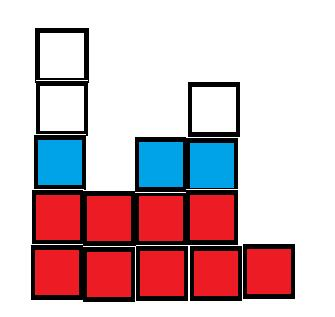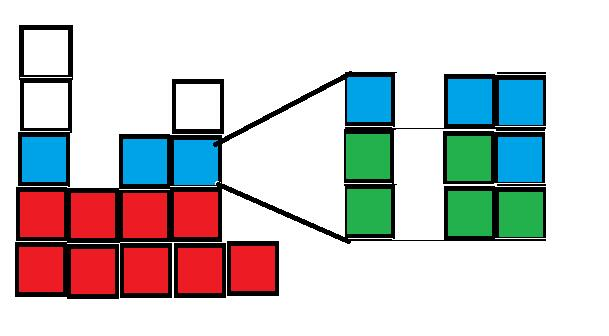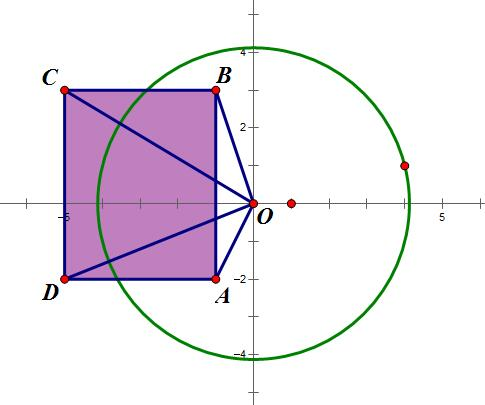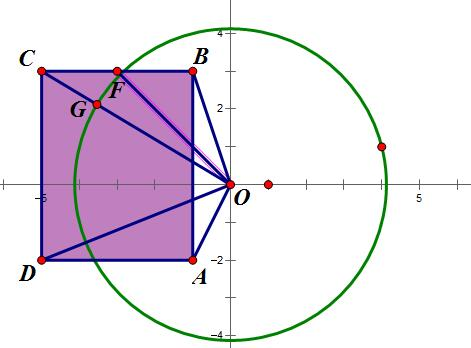# 代码位置：

https://github.com/HarryGuo2012/ACMCode/tree/worldLine/CDOJ/14thPr

# A Graph Problem

## 题解

$$dp[s] = \underset{u,v}{min} (dp[u] + dp[v]),u\cup v = s$$

# Find the Stuff

## 题解# Good Water Problem

## 题解

$$A={p^{a_0},p^{a_1},p^{a_2},\cdots,p^{a_k}}$$

# Happy Shape

## 题解